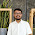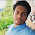## Monday, 12 October 2015

### Program For Tribonacci Series

```import java.util.*;
import java.io.*;
class Tribonacci
{
public static void main(String args[])
{
Scanner sc = new Scanner(System.in);
System.out.print("Enter number till u want Tribonacci series: ");
int n=sc.nextInt();

int a = 0,b = 0,c = 1;
int d = a+b+c;

System.out.println("\nTribonacci Series: ");
System.out.print(a+"\t"+b+"\t"+c);

for(int i=4;i<=n;i++)
{
System.out.print("\t"+d);
a=b;
b=c;
c=d;
d=a+b+c;
}

System.out.println();
}
}
```
```Output:
Enter number till u want Tribonacci series: 10

Tribonacci Series:
0       0       1       1       2       4       7       13      24      44
```

1.Thank u...its wonderful

1.Thanks Soustav Das

2.This comment has been removed by a blog administrator.

3.Can u give the program without using scanner

1.Hello Roy, you want it just to generate without using scanner then you need to have a fixed value for n hard coded.

2.Hello Subhankar Roy, as Leon Manyonho said is absolutely right...and also you can even take a value of n through BufferedReader as well...

3.You can pass the value of n by a method(function) instead of scanner
For example:-
void a(int n)

4.5.This comment has been removed by the author.

6.Thanks for a great post......I created a Tribonacci Series program in C and C++ by taking the above code reference.

7.